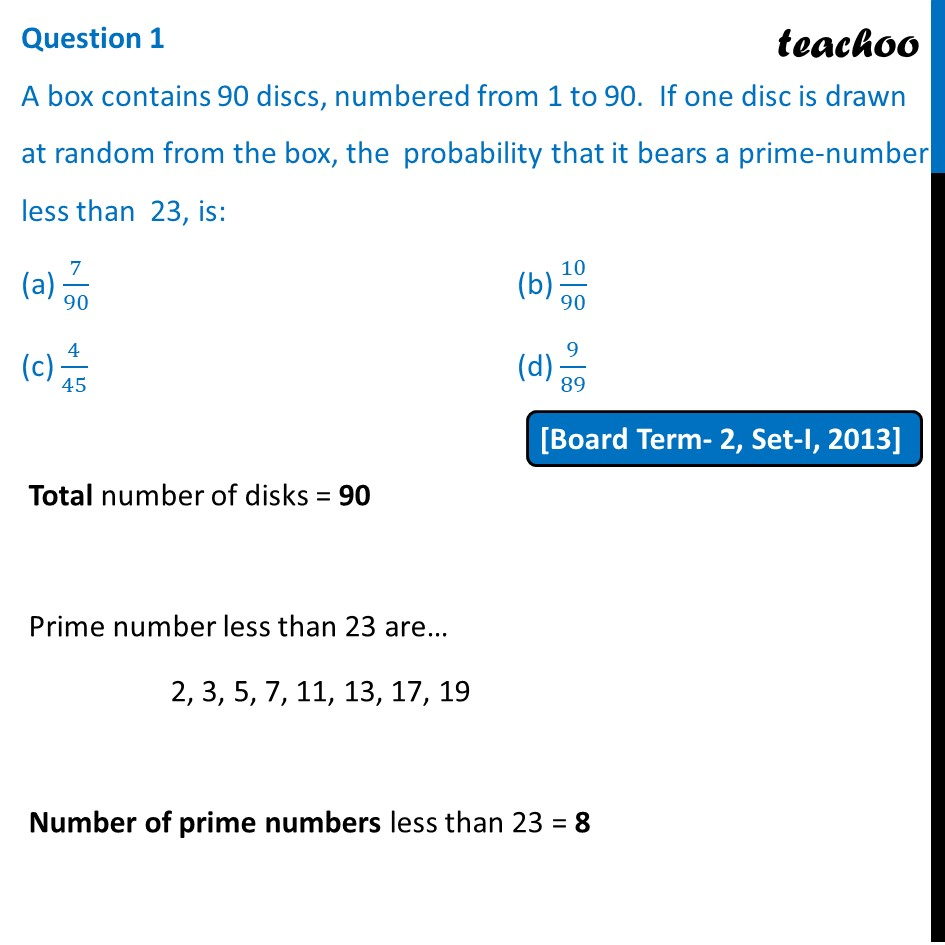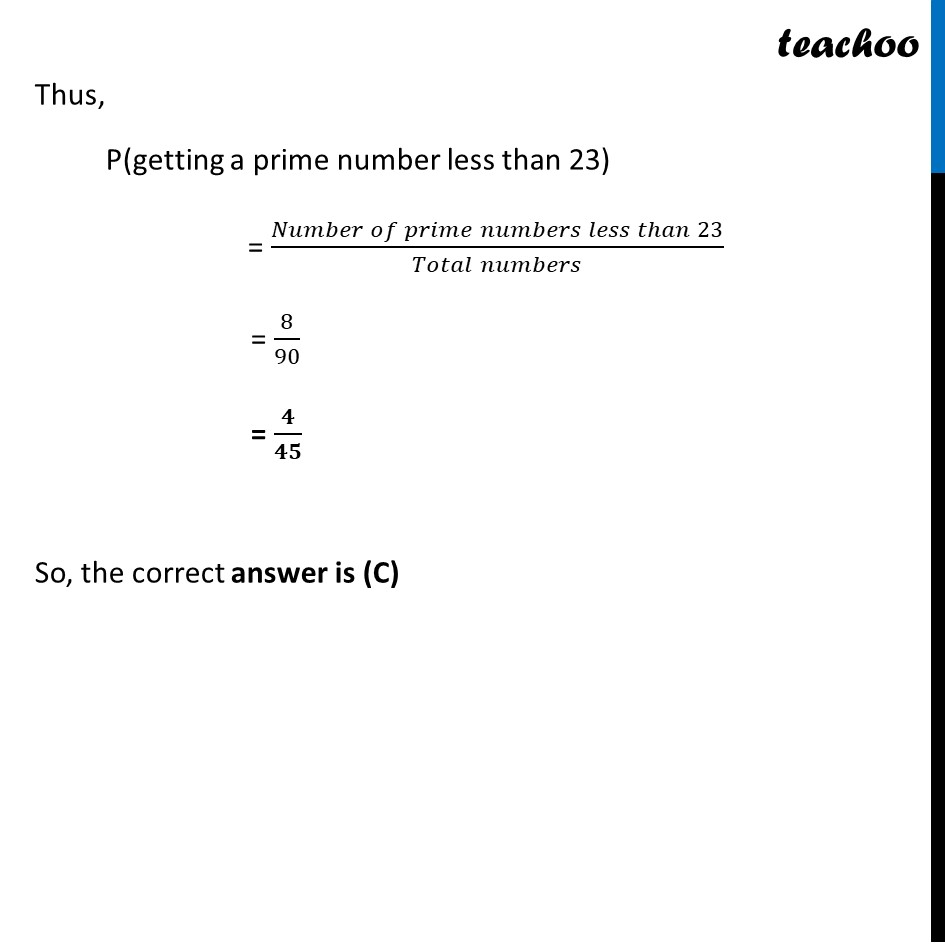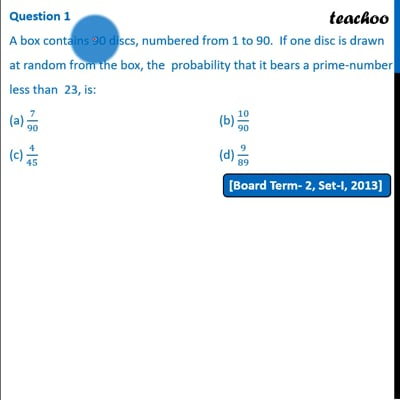Past Year MCQ

Chapter 15 Class 10 Probability
Serial order wise

## (c) 4/45                                                           (d) 9/89This video is only available for Teachoo black users

Introducing your new favourite teacher - Teachoo Black, at only ₹83 per month

### Transcript

Question 1 A box contains 90 discs, numbered from 1 to 90. If one disc is drawn at random from the box, the probability that it bears a prime-number less than 23, is: (a) 7/90 (b) 10/90 (c) 4/45 (d) 9/89 Total number of disks = 90 Prime number less than 23 are… 2, 3, 5, 7, 11, 13, 17, 19 Number of prime numbers less than 23 = 8 Thus, P(getting a prime number less than 23) = (𝑁𝑢𝑚𝑏𝑒𝑟 𝑜𝑓 𝑝𝑟𝑖𝑚𝑒 𝑛𝑢𝑚𝑏𝑒𝑟𝑠 𝑙𝑒𝑠𝑠 𝑡ℎ𝑎𝑛 23)/(𝑇𝑜𝑡𝑎𝑙 𝑛𝑢𝑚𝑏𝑒𝑟𝑠) = 8/90 = 𝟒/𝟒𝟓 So, the correct answer is (C)